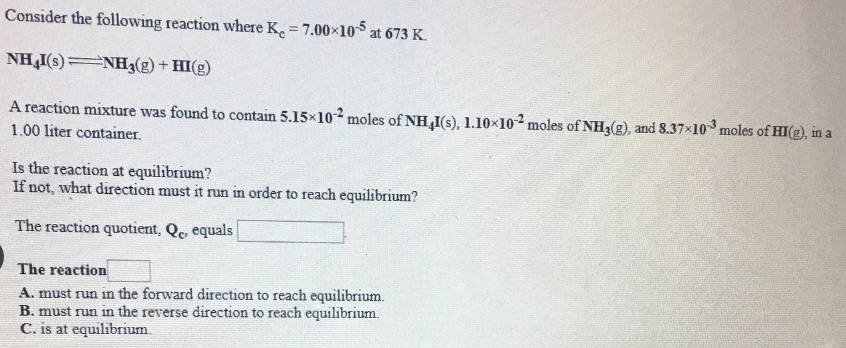# Consider the following reaction where Kc = 7.00X10^-5 at 673 K. NH4I(s) ⇌ NH3(g) + HI(g) A reaction mixture was found to contain 5.15x10^-2 moles of NH4I(s), 1.10x10^-2 moles of NH3(g), and 8.73X10^-3 moles of HI(g), in a 1.00 liter container. Is the reaction at equilibrium? If not, what direction must it run in order to reach equilibrium? The reaction quotient, Qc, equals ____. The reaction ____.